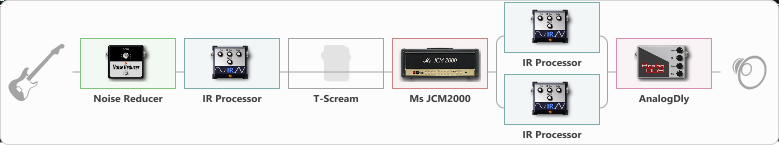# charvel-3

Discussion in 'ToneLib-GFX presets' started by carlinos3, Jun 6, 2021.

1. charvel-3

Preset name: carlos--3

Effects chain:Effect: "Noise Reducer" (Dinámica / Filtro), active - "yes"
{
"Sens" = 92
"Mode" = Soft
}

Effect: "IR Processor" (Gabinetes), active - "yes"
{
"IR" = pre-tonal_dc
"Low Cut (Hz)" = 0
"Hi Cut (kHz)" = 20.0
"Mix" = 67
"Level (dB)" = 18
}

Effect: "T-Scream" (Overdrive / Distorsión), active - "no"
{
"Drive" = 22
"Tone" = 56
"Level" = 42
}

{
"Gain" = 100
"Bass" = 50
"Middle" = 50
"Treble" = 50
"Presence" = 50
"Master" = 60
"Level (dB)" = 0
}

Effect: "Splitter" (Dinámica / Filtro)
{
"A-Bypass" = Off
"A-Pan" = 62
"A-Level" = 55
"B-Bypass" = Off
"B-Pan" = -62
"B-Level" = 55

'A' branch:
{

Effect: "IR Processor" (Gabinetes), active - "yes"
{
"IR" = charv.1_dc
"Low Cut (Hz)" = 0
"Hi Cut (kHz)" = 20.0
"Mix" = 100
"Level (dB)" = 3
}
}
'B' branch:
{

Effect: "IR Processor" (Gabinetes), active - "yes"
{
"IR" = Meshall_V30_MOD_i5 A0
"Low Cut (Hz)" = 0
"Hi Cut (kHz)" = 20.0
"Mix" = 100
"Level (dB)" = 0
}
}
}

Effect: "AnalogDly" (Retrasar), active - "yes"
{
"Time" = 532
"Feedback" = 0
"Tone" = 65
"Mix" = 51
}

Note: You will need to download and install the ToneLib-GFX software to use the preset.

#### Attached Files:

• ###### charvel-3.tlgfx
File size:
75 KB
Views:
2,503
Palomitas likes this.

File size:
165.6 KB
Views:
9